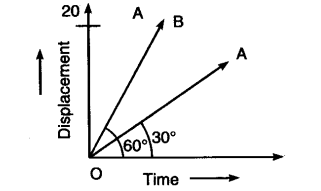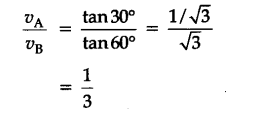# The two straight rays OA and OB on the same displacement-time graph make angle 30° and 60° with time axis respectively as shown in figure:

The two straight rays OA and OB on the same displacement-time graph make angle 30° and 60° with time axis respectively as shown in figure:
(i) Which ray represents greater velocity ?
(ii) What is the ratio of two velocity represented by OA and OB ?Since the slope of the displacement-time graph of uniform motion in one dimention represents the velocity of the object, hence the line showing greater slope in graph corresponds to greater velocity of the object. Therefore OB represents greater velocity.
(ii) Ratio of two velocities1 Like